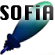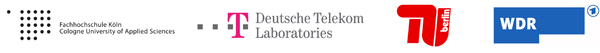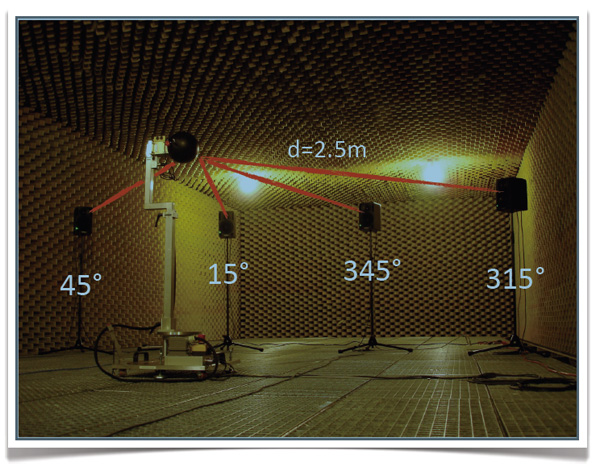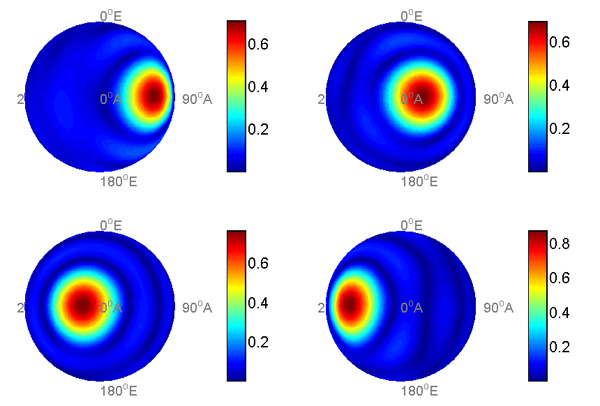Welcome to SOFiA
Who is behind SOFiA
Feature overview
System overview
Function reference
mergeArrayData
F/D/T
gauss
lebedev
S/W/G
S/T/C
W/G/C
S/F/E
M/F
R/F/I
P/D/C
I/T/C
makeMTX
makeIR
visual3D
Coordinate System
Application Examples
Example 1
Example 2
Example 3
Example 4
Example 5
Example 6
Example 7
Example 8
Array Datasets
VariSphear system
Groups and Mailinglists
Contact and Support
How to Reference

# SOFiA application example 5

This example shows 4 "plane waves" impinging to a real array in a rigid sphere configuration that arrive at different times. We work on a real array dataset: EXAMPLE 3. The four sources have the same level but different time delays (45°:0ms, 15°:16ms, 345°:32ms, 315°:48ms). This example shows how to get access to the spatiotemporal sound field information running a plane wave decomposition on different time windows.## File(s)

Run `sofiaAE5.m`.

Locate the folder `EXAMPLE3_SpatioTemporal` containing the required array data.

## OutputTake care: This figure shows frontal views of the array response and the photo on top shows the rear side of the array.

## Code

 ```/* % SOFiA example 5: Spatiotemporal resolution % SOFiA Version : R11-1220 % Array Dataset : R11-1018 clear all clc % Read VariSphear dataset % !!! LOCATE THE FOLDER: "EXAMPLE2_LevelResolution" timeData = sofia_readVSAdata(); ``` ` ` ` ` ``` figure(2) % Enable to get an IR overview clf(); area(timeData.irOverlay'); fftOversize = 2; startSample = [400 1100 1800 2500]; % Examplary values (Enable area plot) blockSize = 256; figure(1) clf(); for ctr=1:size(startSample, 2) % Here we have simply put a loop around, because this is good for % understanding this experiment. The loop content can easily be optimized. % Transform time domain data to frequency domain and generate kr-vector [fftData, kr, f] = sofia_fdt(timeData, fftOversize, startSample(ctr), startSample(ctr)+blockSize); % Spatial Fourier Transform Nsft = 5; Pnm = sofia_stc(Nsft, fftData, timeData.quadratureGrid); ``` ``` % Radial Filters for a rigid sphere array Nsft = 5; Pnm = sofia_stc(Nsft, fftData, timeData.quadratureGrid); % Radial filters for a rigid sphere array Nrf = Nsft; % radial filter order maxAmp = 10; % Maximum modal amplification in [dB] ac = 2; % Array configuration: 2 0 Rigid Sphere dn = sofia_mf(Nrf, kr, ac, maxAmp); % radial filters % Make MTX Nmtx = Nsft; krIndex = 90; % Choose the kr-bin (Frequency) to display. mtxData = sofia_makeMTX(Nmtx, Pnm, dn, krIndex); subplot(2, 2, ctr); sofia_visual3D(mtxData, 0); view(90, 0) end disp(The plot shows the response at a frequency of ',num2str(round(10*f(krIndex))/10),'Hz'); ``` `*/`